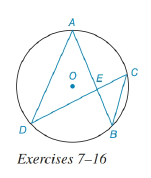Chapter 6.3, Problem 7E### Elementary Geometry for College St...

6th Edition
Daniel C. Alexander + 1 other
ISBN: 9781285195698

#### Solutions

Chapter
Section### Elementary Geometry for College St...

6th Edition
Daniel C. Alexander + 1 other
ISBN: 9781285195698
Textbook Problem
1 views

# In the figure for Exercises 7 to 16, O is the center of the circle. See Theorem 6.3.5.Given: A E = 6 , E B = 4 , D E = 8 Find: EC.To determine

To find:

To find EC.

Explanation

Given that AE=6,EB=4,DE=8.

The diagrammatic representation is given below,

Theorem:

If two chords intersect within a circle, then the product of the lengths of the segments (parts) of one chord is equal to the product of the lengths of the segments of the other chord.

By using the theorem to get the following,

AEEB=DEEC

Substitute the values AE=6,EB=4,DE=8 in the above equation to get the following,

### Still sussing out bartleby?

Check out a sample textbook solution.

See a sample solution

#### The Solution to Your Study Problems

Bartleby provides explanations to thousands of textbook problems written by our experts, many with advanced degrees!

Get Started

#### Find the x- and y-intercepts of the graph of y=x22x3.

Calculus: An Applied Approach (MindTap Course List)

#### converges to: 2 the series diverges

Study Guide for Stewart's Multivariable Calculus, 8th

#### Find for x = 3t2 + 1, y = t6 + 6t5. t4 + 5t3 4t3 + 15t2

Study Guide for Stewart's Single Variable Calculus: Early Transcendentals, 8th